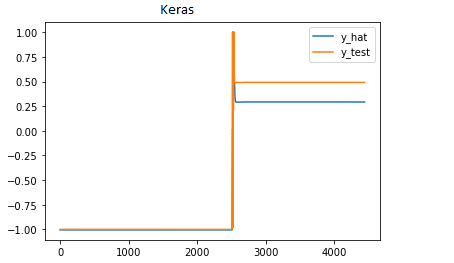# Convert Keras LSTM to PyTorch LSTM

Hello everyone,
I have been working on converting a Keras LSTM time-series prediction model into PyTorch for a project I am working on. I am new to PyTorch and have been using this as a chance to get familiar with it. I have implemented a model based on what I can find on my own, but the outputs do not compare like I was expecting. I expect some variation due to random weight initialization but not this much.

The Keras model implements some early stopping, which I have not done in PyTorch. I’m hoping to rule out any model issues before going down that rabbit hole.

In short, I am trying to implement what looks like a 2-layer LSTM network with a full-connected, linear output layer.

Both LSTM layers have the same number of features (80). I believe PyTorch LSTM dropout does not apply to the last layer which is slightly different from the Keras model that has a drop out after each LSTM layer. However, I’ve removed all drop out and got similar, mismatched results.

I am reasonably confident in my handling of input shapes and ‘many-to-one’ down-selecting with my linear layer, but nothing is off the table at this point.

My X_train data set is size (454, 250, 25), for 454 samples of 250 time steps with 25 features.
My y_train data set is size (454,1), for 454 samples of 1 target feature.
Batch size is 64 samples.

Here is the Keras model I am trying to match. I have replaced variable parameters with numerical values to improve readability between code segments.

Keras imports:

``````    from keras.models import Sequential, load_model
from keras.callbacks import History, EarlyStopping, Callback
from keras.layers.recurrent import LSTM
from keras.layers.core import Dense, Activation, Dropout
import numpy as np
import os
import logging
``````

Following Keras model is wrapped in a class with the following definition:

``````  cbs = [History(), EarlyStopping(monitor='val_loss',
patience=10,
min_delta=0.0003,
verbose=0)]

self.model = Sequential()

self.model.compile(loss='mse',

self.model.fit(X_train,
y_train,
batch_size=64,
epochs=35,
validation_split=0.2,
callbacks=cbs,
verbose=True)
This text will be hidden
``````

PyTorch attempt:

``````import numpy as np
import os
import logging
import torch
import torch.nn as nn
import torch.optim as optim
from torch.utils.data import DataLoader, Dataset, TensorDataset
from torch.utils.data.dataset import random_split
from sklearn.model_selection import train_test_split

class LSTM_PT(nn.Module):
def __init__(self, n_features =25, hidden_dims = [80,80], seq_length = 250, batch_size=64, n_predictions=1, device = torch.device("cuda:0"), dropout=0.3):
super(LSTM_PT, self).__init__()

self.n_features = n_features
self.hidden_dims = hidden_dims
self.seq_length = seq_length
self.num_layers = len(self.hidden_dims)
self.batch_size = batch_size
self.device = device

print(f'number of layers :{self.num_layers}')

self.lstm1 = nn.LSTM(
input_size = n_features,
hidden_size = hidden_dims,
batch_first = True,
dropout = dropout,
num_layers = self.num_layers)

self.linear = nn.Linear(self.hidden_dims, n_predictions)

self.hidden = (
torch.randn(self.num_layers, self.batch_size, self.hidden_dims).to(self.device),
torch.randn(self.num_layers, self.batch_size, self.hidden_dims).to(self.device)
)

def init_hidden_state(self):
#initialize hidden states (h_n, c_n)

self.hidden = (
torch.randn(self.num_layers, self.batch_size, self.hidden_dims).to(self.device),
torch.randn(self.num_layers, self.batch_size, self.hidden_dims).to(self.device)
)

def forward(self, sequences):

batch_size, seq_len, n_features = sequences.size() #batch_first

# LSTM inputs: (input, (h_0, c_0))
#input of shape (seq_len, batch, input_size)....   input_size = num_features
#or (batch, seq_len, input_size) if batch_first = True

lstm1_out , (h1_n, c1_n) = self.lstm1(sequences, (self.hidden, self.hidden)) #hidden = h_n, hidden = c_n

#Output: output, (h_n, c_n)
#output is of shape (batch_size, seq_len, hidden_size) with batch_first = True

last_time_step = lstm1_out[:,-1,:] #lstm_out[:,-1,:] or h_n[-1,:,:]
y_pred = self.linear(last_time_step)
#output is shape (N, *, H_out)....this is (batch_size, out_features)

return y_pred

def initialize_weights(model):
if type(model) in [nn.Linear]:
nn.init.xavier_uniform_(model.weight.data)
elif type(model) in [nn.LSTM, nn.RNN, nn.GRU]:
nn.init.xavier_uniform_(model.weight_hh_l0)
nn.init.xavier_uniform_(model.weight_ih_l0)

def predict_model(model, data, batch_size, device):

print('Starting predictions...')

y_hat_batch = model(X_batch)
y_hat = torch.cat([y_hat, y_hat_batch])

y_hat = torch.flatten(y_hat[batch_size:,:]).cpu().numpy() #y_hat[batchsize:] is to remove first empty 'section'
print('Predictions complete...')
return y_hat

def train_model( model, train_data, train_labels, test_data, test_labels, batch_size, num_epochs, device):

model.apply(initialize_weights)

training_losses = []
validation_losses = []

loss_function = nn.MSELoss()

train_hist = np.zeros(num_epochs)

X_train, X_validation, y_train, y_validation = train_test_split(train_data, train_labels, train_size=0.8)

train_dataset=TensorDataset(X_train,y_train)
validation_dataset=TensorDataset(X_validation,y_validation)

model.train()

print("Beginning model training...")

for t in range(num_epochs):
train_losses_batch = []

y_hat_train = model(X_batch_train)
loss = loss_function(y_hat_train.float(), y_batch_train)
train_loss_batch = loss.item()
loss.backward()
optimizer.step()
train_losses_batch.append(train_loss_batch)

training_loss = np.mean(train_losses_batch)
training_losses.append(training_loss)

val_losses_batch = []
model.eval()
y_hat_val = model(X_val_batch)
val_loss_batch = loss_function(y_hat_val.float(), y_val_batch).item()
val_losses_batch.append(val_loss_batch)
validation_loss = np.mean(val_losses_batch)
validation_losses.append(validation_loss)

print(f"[{t+1}] Training loss: {training_loss} \t Validation loss: {validation_loss} ")

print('Training complete...')
return model.eval()
``````

Keras results (target):

y_hat is model output and y_test is test data.My PyTorch output (y_hat) is similar in shape but plateaus at ~-0.5. I would post the image but new users can only post oneI’ve gone down the rabbit hole of differences between Keras and PyTorch and learned about how each package initializes weights differently. I’ve changed my code to use the same initialization methods and got MUCH better results.

Anyone who gets different results between the packages when their model looks correct should try this.

1 Like